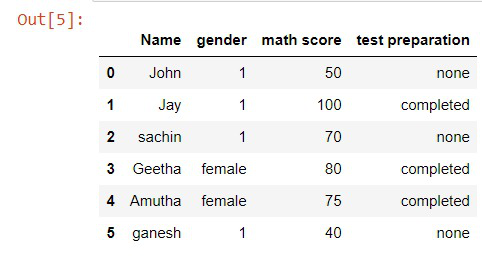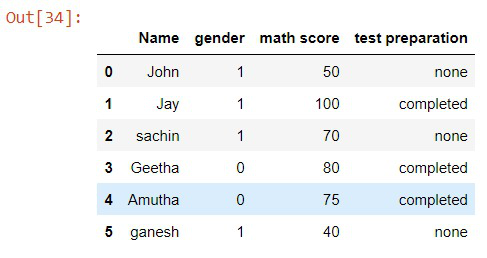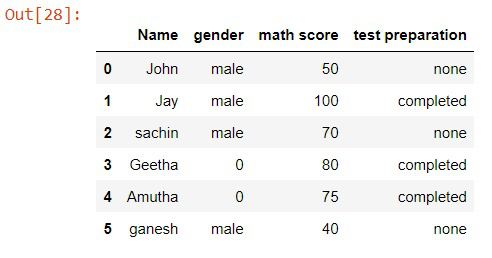Open in App
Not now

# How to Replace Values in Column Based on Condition in Pandas?

• Difficulty Level : Medium
• Last Updated : 28 Nov, 2021

In this article, we are going to discuss the various methods to replace the values in the columns of a dataset in pandas with conditions. This can be done by many methods lets see all of those methods in detail.

## Method 1 : Using  dataframe.loc[] function

With this method, we can access a group of rows or columns with a condition or a boolean array. If we can access it we can also manipulate the values, Yes! this is our first method by the dataframe.loc[] function in pandas we can access a column and change its values with a condition.

Now, we are going to change all the “male” to 1 in the gender column.

Syntax: df.loc[ df[“column_name”] == “some_value”, “column_name”] = “value”

some_value = The value that needs to be replaced

value = The value that should be placed instead.

Note: You can also use other operators to construct the condition to change numerical values..

## Python3

 `# Importing the libraries``import` `pandas as pd``import` `numpy as np`` ` `# data``Student ``=` `{``    ``'Name'``: [``'John'``, ``'Jay'``, ``'sachin'``, ``'Geetha'``, ``'Amutha'``, ``'ganesh'``],``    ``'gender'``: [``'male'``, ``'male'``, ``'male'``, ``'female'``, ``'female'``, ``'male'``],``    ``'math score'``: [``50``, ``100``, ``70``, ``80``, ``75``, ``40``],``    ``'test preparation'``: [``'none'``, ``'completed'``, ``'none'``, ``'completed'``,``                         ``'completed'``, ``'none'``],``}`` ` `# creating a Dataframe object``df ``=` `pd.DataFrame(Student)`` ` `# Applying the condition``df.loc[df[``"gender"``] ``=``=` `"male"``, ``"gender"``] ``=` `1`

Output:## Method 2: Using NumPy.where() function

Another method we are going to see is with the NumPy library. NumPy is a very popular library used for calculations with 2d and 3d arrays. It gives us a very useful method where() to access the specific rows or columns with a condition. We can also use this function to change a specific value of the columns.

This numpy.where() function should be written with the condition followed by the value if the condition is true and a value if the condition is false. Now, we are going to change all the “female” to 0 and “male” to 1 in the gender column.

syntax: df[“column_name”] = np.where(df[“column_name”]==”some_value”, value_if_true, value_if_false)

## Python3

 `# Importing the libraries``import` `pandas as pd``import` `numpy as np`` ` `# data``student ``=` `{``    ``'Name'``: [``'John'``, ``'Jay'``, ``'sachin'``, ``'Geetha'``, ``'Amutha'``, ``'ganesh'``],``    ``'gender'``: [``'male'``, ``'male'``, ``'male'``, ``'female'``, ``'female'``, ``'male'``],``    ``'math score'``: [``50``, ``100``, ``70``, ``80``, ``75``, ``40``],``    ``'test preparation'``: [``'none'``, ``'completed'``, ``'none'``, ``'completed'``,``                         ``'completed'``, ``'none'``],``}`` ` `# creating a Dataframe object``df ``=` `pd.DataFrame(student)`` ` ` ` `# Applying the condition``df[``"gender"``] ``=` `np.where(df[``"gender"``] ``=``=` `"female"``, ``0``, ``1``)`

Output:## Method 3: Using pandas masking function

Pandas masking function is made for replacing the values of any row or a column with a condition. Now using this masking condition we are going to change all the “female” to 0 in the gender column.

syntax: df[‘column_name’].mask( df[‘column_name’] == ‘some_value’, value , inplace=True )

## Python3

 `# Importing the libraries``import` `pandas as pd``import` `numpy as np`` ` `# data``student ``=` `{``    ``'Name'``: [``'John'``, ``'Jay'``, ``'sachin'``, ``'Geetha'``, ``'Amutha'``, ``'ganesh'``],``    ``'gender'``: [``'male'``, ``'male'``, ``'male'``, ``'female'``, ``'female'``, ``'male'``],``    ``'math score'``: [``50``, ``100``, ``70``, ``80``, ``75``, ``40``],``    ``'test preparation'``: [``'none'``, ``'completed'``, ``'none'``, ``'completed'``, ``                         ``'completed'``, ``'none'``],``}`` ` `# creating a Dataframe object``df ``=` `pd.DataFrame(student)`` ` `# Applying the condition``df[``'gender'``].mask(df[``'gender'``] ``=``=` `'female'``, ``0``, inplace``=``True``)`` ` `# Try this too``#df['math score'].mask(df['math score'] >=60 ,'good', inplace=True)`

Output:My Personal Notes arrow_drop_up